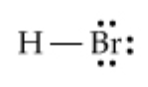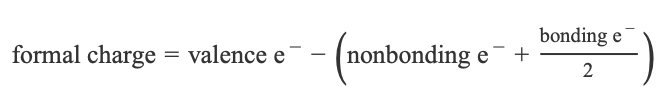# Problem: What is the formal charge on the bromine atom in HBr? Use the periodic table as needed.Express the formal charge numerically (e.g., +1).The Lewis structure of HBr is shown here:The formula for formal chargeThe formal charge of an atom in a molecule can be calculated based on the following formula:

###### FREE Expert Solution
85% (472 ratings)View Complete Written Solution
###### Problem Details

What is the formal charge on the bromine atom in HBr? Use the periodic table as needed.

Express the formal charge numerically (e.g., +1).

The Lewis structure of HBr is shown here:The formula for formal charge

The formal charge of an atom in a molecule can be calculated based on the following formula: